English
English
Russian
French
Chinese
Spanish
// Blog May 7, 2019

# What is the Difference Between Clo, Tog, Thermal Resistance, and Thermal Conductivity?

Textiles and textile product manufacturers have for decades looked to physical tests to quantify thermal performance of their products. Many units are used in these applications – varying from country to country, lab to lab, and within different application spaces. This can lead to confusion in comparing values between thermal unit systems. This article aims to define some of these units and to provide conversion factors to aid in apples-to-apples thermal insulation property comparisons, using the thermal conductivity as the starting point.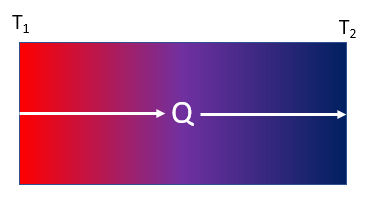Thermal conductivity, k (in W/mK), is the property which governs how much heat, Q (in W), can flow through a defined cross-section, A (in m2), and thickness, x (in m), of a material under a certain temperature gradient. In a one-dimensional case, this situation is dictated by the equation below: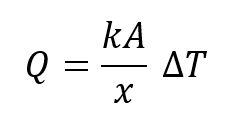Thermal resistance, R (in m2K/W) is the property defining the resistance to heat transfer of a material with a defined thickness, x. It is related to thermal conductivity with the following equation: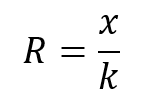Clo and togs are units unique to the textiles industry, and provide other ways of representing the thermal resistance of a textile or textile product. The three units are related by the following equation: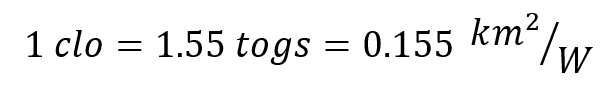If you know the thickness of the material, you can convert between thermal conductivity, thermal resistance, clo, and togs with the following equation: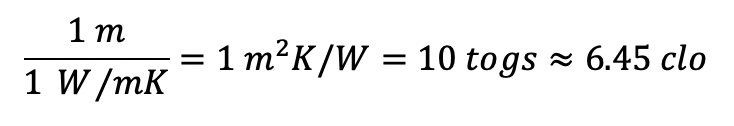For example, a blanket/bedding material 4 mm thick with a thermal conductivity of 0.028 W/mK has a thermal resistance described by the following equation: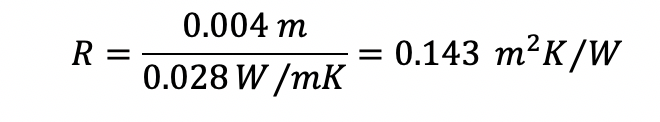Which, using the conversion formula above, we can determine is equivalent to 1.43 togs or 0.921 clo.